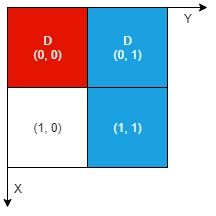##### Welcome to Subscribe On Youtube

Formatted question description: https://leetcode.ca/all/2201.html

# 2201. Count Artifacts That Can Be Extracted (Medium)

There is an n x n 0-indexed grid with some artifacts buried in it. You are given the integer n and a 0-indexed 2D integer array artifacts describing the positions of the rectangular artifacts where artifacts[i] = [r1i, c1i, r2i, c2i] denotes that the ith artifact is buried in the subgrid where:

• (r1i, c1i) is the coordinate of the top-left cell of the ith artifact and
• (r2i, c2i) is the coordinate of the bottom-right cell of the ith artifact.

You will excavate some cells of the grid and remove all the mud from them. If the cell has a part of an artifact buried underneath, it will be uncovered. If all the parts of an artifact are uncovered, you can extract it.

Given a 0-indexed 2D integer array dig where dig[i] = [ri, ci] indicates that you will excavate the cell (ri, ci), return the number of artifacts that you can extract.

The test cases are generated such that:

• No two artifacts overlap.
• Each artifact only covers at most 4 cells.
• The entries of dig are unique.

Example 1:Input: n = 2, artifacts = [[0,0,0,0],[0,1,1,1]], dig = [[0,0],[0,1]]
Output: 1
Explanation:
The different colors represent different artifacts. Excavated cells are labeled with a 'D' in the grid.
There is 1 artifact that can be extracted, namely the red artifact.
The blue artifact has one part in cell (1,1) which remains uncovered, so we cannot extract it.
Thus, we return 1.


Example 2:Input: n = 2, artifacts = [[0,0,0,0],[0,1,1,1]], dig = [[0,0],[0,1],[1,1]]
Output: 2
Explanation: Both the red and blue artifacts have all parts uncovered (labeled with a 'D') and can be extracted, so we return 2.


Constraints:

• 1 <= n <= 1000
• 1 <= artifacts.length, dig.length <= min(n2, 105)
• artifacts[i].length == 4
• dig[i].length == 2
• 0 <= r1i, c1i, r2i, c2i, ri, ci <= n - 1
• r1i <= r2i
• c1i <= c2i
• No two artifacts will overlap.
• The number of cells covered by an artifact is at most 4.
• The entries of dig are unique.

Companies:
IMC

Related Topics:
Array, Hash Table

Similar Questions:

## Solution 1.

Create an NxN array M. M[i][j] = true if the cell (i, j) is digged.

For each artifact, traverse all its cells. If all the cells are digged, increment the answer.

// OJ: https://leetcode.com/problems/count-artifacts-that-can-be-extracted/
// Time: O(DIG + N^2)
// Space: O(N^2)
class Solution {
public:
int digArtifacts(int n, vector<vector<int>>& A, vector<vector<int>>& dig) {
vector<vector<bool>> M(n, vector<bool>(n)); // M[i][j] = true if it's digged.
for (auto &d : dig) M[d][d] = true;
int ans = 0;
for (auto &v : A) {
bool good = true;
for (int x = v; x <= v && good; ++x) {
for (int y = v; y <= v && good; ++y) {
if (!M[x][y]) good = false;
}
}
if (good) ++ans;
}
return ans;
}
};


## Discussion

https://leetcode.com/problems/count-artifacts-that-can-be-extracted/discuss/1844106